# Precalculus : Find the Norm of a Vector

## Example Questions

### Example Question #1 : Find The Norm Of A Vector

Find the norm of the vector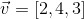.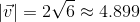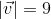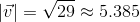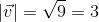Explanation:

We find the norm of a vector by finding the sum of each element squared and then taking the square root.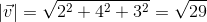.

### Example Question #2 : Find The Norm Of A Vector

Find the norm of the vector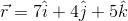.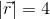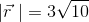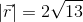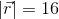Explanation:

We find the norm of a vector by finding the sum of each component squared and then taking the square root of that sum.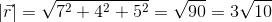### Example Question #3 : Find The Norm Of A Vector

Find the norm of the vector: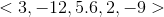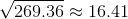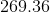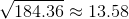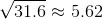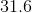Explanation:

The norm of a vector is also known as the length of the vector. The norm is given by the formula: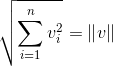.

Here, we have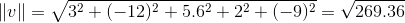,

### Example Question #4 : Find The Norm Of A Vector

Find the norm of vector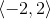.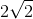Explanation:

Write the formula to find the norm, or the length the vector.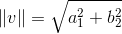Substitute the known values of the vector and solve.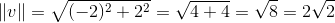### Example Question #5 : Find The Norm Of A Vector

Find the norm (magnitude) of the following vector: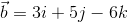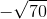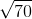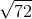Explanation:

Use the following equation to find the magnitude of a vector: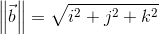In this case we have:So plug in our values: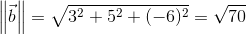So: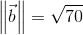### All Precalculus Resources